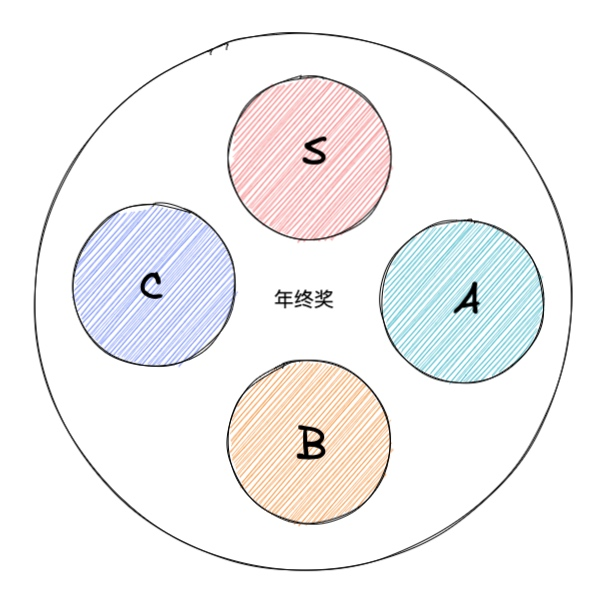# 策略模式### 实现策略模式``````function getBonus(level, salary) {
if (level === 'S') {
return salary * 4
} else if (level === 'A') {
return salary * 3
} else if (level === 'B') {
return salary * 2
} else if (level === 'C') {
return salary * 1
}
}
``````

1. 既有判断等级的逻辑又有计算奖金的逻辑，违反了单一职责；
2. 若哪天需要加另一个等级 D 需要直接在函数里面修改，违反了开闭原则；
3. 如果需要在别的地方使用其中一个等级，只能 C / V 大法，没有复用性。

1. 拆分
``````function sLevel(salary) {
return salary * 4
}

function aLevel(salary) {
return salary * 3
}

function bLevel(salary) {
return salary * 2
}

function cLevel(salary) {
return salary * 1
}

function getBonus(level, salary) {
if (level === 'S') {
return sLevel(salary)
} else if (level === 'A') {
return aLevel(salary)
} else if (level === 'B') {
return bLevel(salary)
} else if (level === 'C') {
return cLevel(salary)
}
}

getBonus('S',1000)
``````

``````
let strategies = {
'S': function (salary) {
return salary * 4
},
'A': function (salary) {
return salary * 3
},
'B': function (salary) {
return salary * 2
},
'C': function (salary) {
return salary * 1
}
}

function getBonus(level, salary) {
return strategies[level](salary)
}

getBonus('S',1000)
``````

``````strategies.D = function (salary) {
return salary * 0.9
}
``````